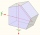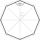# Polygon + sine - math problems

#### Number of problems found: 16

• PentadecagonCalculate the content of a regular 15-sides polygon inscribed in a circle with radius r = 4. Express the result to two decimal places.
• Regular n-gonWhich regular polygon have a radius of circumscribed circle r = 10 cm and the radius of inscribed circle p = 9.962 cm?
• PentagonCalculate the area of a regular pentagon, which diagonal is u=16.
• Inscribed and described circleFind the radii of a circle inscribed and circumscribed by a regular pentagon whose side measures 3 cm.
• Hexagon 5The distance of parallel sides of regular hexagonal is 61 cm. Calculate the length of the radius of the circle described to this hexagon.
• KitesBoys run kite on a cable of 68 meters long. What is the kite altitude, if the angle from the horizontal plane is 72°?
• Hexagon ACalculate area of regular hexagon inscribed in circle with radius r=9 cm.
• n-gonWhat is the side length of the regular 5-gon inscribed in a circle of radius 12 cm?
• DecagonCalculate the area and circumference of the regular decagon when its radius of a circle circumscribing is R = 1m
• Regular 5-gonCalculate the area of the regular pentagon with side 7 cm.
• Nine-gonCalculate the perimeter of a regular nonagon (9-gon) inscribed in a circle with a radius 13 cm.
• The mastA 40 m high mast is secured in half by eight ropes of 25 m long. The ends of the ropes are equidistant from each other. Calculate this distance.
• PentagonCalculate the length of side, circumference and area of a regular pentagon, which is inscribed in a circle with radius r = 6 cm.
• Diagonals of pentagonCalculate the diagonal length of the regular pentagon: a) inscribed in a circle of radius 12dm; b) a circumscribed circle with a radius of 12dm.
• Inner anglesThe inner angles of the triangle are 30°, 45° and 105° and its longest side is 10 cm. Calculate the length of the shortest side, write the result in cm up to two decimal places.
• Pentagonal prismThe regular pentagonal prism is 10 cm high. The radius of the circle of the described base is 8 cm. Calculate the volume and surface area of the prism.

Do you have an interesting mathematical word problem that you can't solve it? Submit a math problem, and we can try to solve it.

We will send a solution to your e-mail address. Solved examples are also published here. Please enter the e-mail correctly and check whether you don't have a full mailbox.

Please do not submit problems from current active competitions such as Mathematical Olympiad, correspondence seminars etc...
Most natural application of trigonometry and trigonometric functions is a calculation of the triangles. Common and less common calculations of different types of triangles offers our triangle calculator. Word trigonometry comes from Greek and literally means triangle calculation. Polygon Problems. Sine - math problems.